# Class 10 NCERT Solutions- Chapter 7 Coordinate Geometry – Exercise 7.3

• Last Updated : 04 Mar, 2021

### (i) (2, 3), (-1, 0), (2, -4)

Solution:

Area = 1/2 [x1(y2 – y3) + x2(y3 – y1) + x3(y1 – y2)]

Attention reader! All those who say programming isn't for kids, just haven't met the right mentors yet. Join the  Demo Class for First Step to Coding Coursespecifically designed for students of class 8 to 12.

The students will get to learn more about the world of programming in these free classes which will definitely help them in making a wise career choice in the future.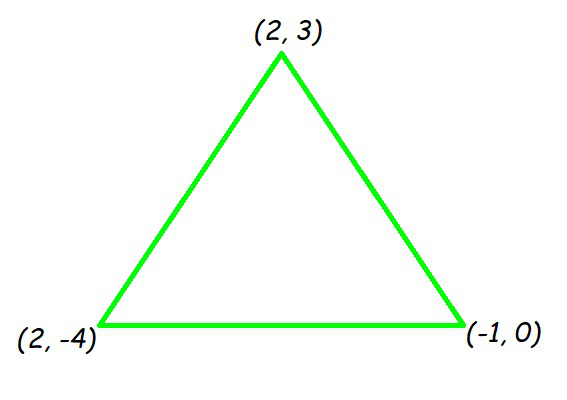Now by putting all the values in the formula, we will get

Area of triangle = 1/2 [2(0 – (-4)) + (-1)((-4) – (3)) + 2(3 – 0)]

= 1/2 [8 + 7 + 6]

= 21/2

So, the area of triangle is 21/2 square units.

### (ii) (-5, -1), (3, -5), (5, 2)

Solution:

Area = 1/2 [x1(y2 – y3) + x2(y3 – y1) + x3(y1 – y2)]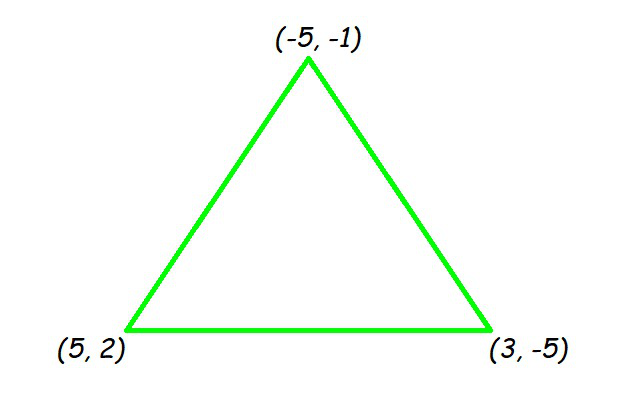Area of the triangle = 1/2 [(-5)((-5) – (2)) + 3(2 – (-1)) + 5((-1) – (-5))]

= 1/2[35 + 9 + 20]

= 32

So, the area of the triangle is 32 square units.

### (i) (7, -2), (5, 1), (3, -k)

Solution:

As we know the result, for collinear points, area of triangle formed by them is always zero.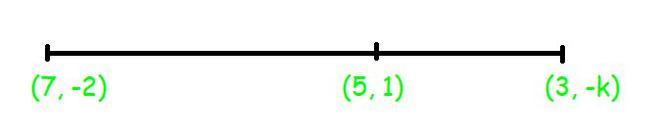Let points (7, -2) (5, 1), and (3, k) are vertices of a triangle. (As given in the question)

Area of triangle = 1/2 [7(1 – k) + 5(k – (-2)) + 3((-2) – 1)] = 0

7 – 7k + 5k +10 -9 = 0

-2k + 8 = 0

k = 4

### (ii) (8, 1), (k, -4), (2, -5)

Solution:

As we know the result, for collinear points, area of triangle formed by them is zero.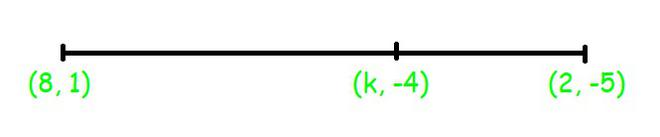So we can say that for points (8, 1), (k, – 4), and (2, – 5), area = 0

1/2 [8((-4) – (-5)) + k((-5) – (1)) + 2(1 -(-4))] = 0

8 – 6k + 10 = 0

6k = 18

k = 3

### Question 3. Find the area of the triangle formed by joining the mid-points of the sides of the triangle whose vertices are (0, -1), (2, 1), and (0, 3). Find the ratio of this area to the area of the given triangle.

Solution:

Let us assume that vertices of the triangle be A(0, -1), B(2, 1), C(0, 3).

Let us assume that D, E, F be the mid-points of the sides of triangle.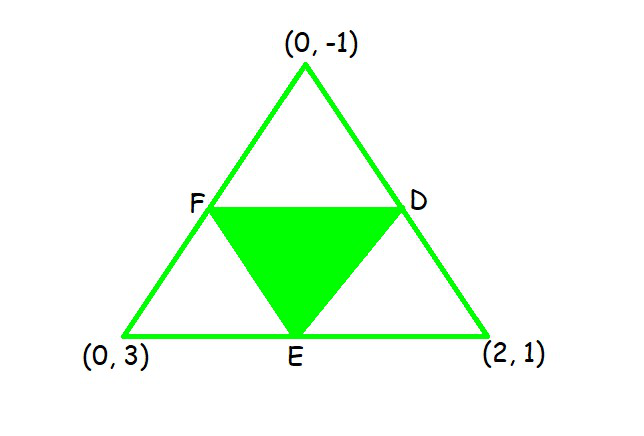Coordinates of D, E, and F are

D = ((0 + 2)/2, (-1 + 1)/2) = (1, 0)

E = ((0+ 0)/2, (-1 + 3)/2) = (0, 1)

F = ((2+0)/2, (1 + 3)/2) = (1, 2)

Area(ΔDEF) = 1/2 [1(2 – 1) + 1(1 – 0) + 0(0 – 2)]  = 1/2 (1+1) = 1

Area of ΔDEF is 1 square units

Area(ΔABC) = 1/2 [0(1 – 3) + 2(3 – (-1)) + 0((-1) – 1)] = 1/2  = 4

Area of ΔABC is 4 square units

So, the required ratio is 1:4.

### Question 4. Find the area of the quadrilateral whose vertices, taken in order, are (-4, -2), (-3, -5), (3, -2), and (2, 3).

Solution:

Let the vertices of the quadrilateral be A(-4, -2), B(-3, -5), C(3, -2), and D(2, 3).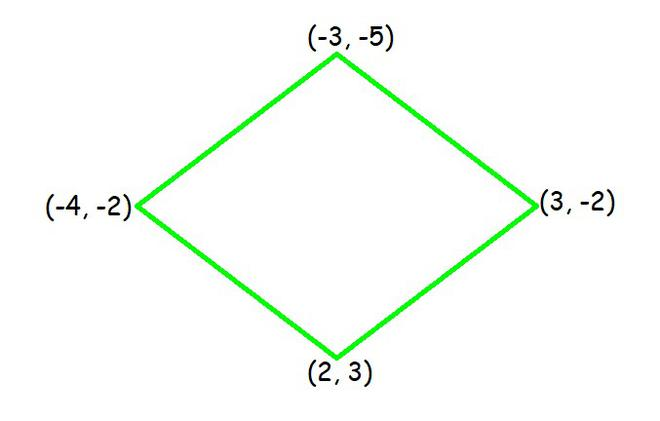Here, AC divide quadrilateral into two triangles.

Now, we have two triangles ΔABC and ΔACD.

Area of ΔABC = 1/2 [(-4)((-5) – (-2)) + (-3)((-2) – (-2)) + 3((-2) – (-5))]

= 1/2 [12 + 0 + 9]

= 21/2 square units

Area of ΔACD = 1/2 [(-4)((-2) – (3)) + 3((3) – (-2)) + 2((-2) – (-2))]

= 1/2 [20 + 15 + 0]

= 35/2 square units

Now we will add area of both triangle and resultant will give area of quadrilateral

Area of quadrilateral ABCD = Area of ΔABC + Area of ΔACD

= (21/2 + 35/2) square units = 28 square units

### Question 5. You have studied in Class IX that a median of a triangle divides it into two triangles of equal areas. Verify this result for ΔABC whose vertices are A (4, -6), B (3, -2), and C (5, 2).

Solution:

Let the vertices of the triangle be A(4, -6), B(3, -2), and C(5, 2).

Let us assume that D be the mid-point of side BC of ΔABC. So, AD is the median in ΔABC.

Coordinates of point D = Midpoint of BC = (4, 0)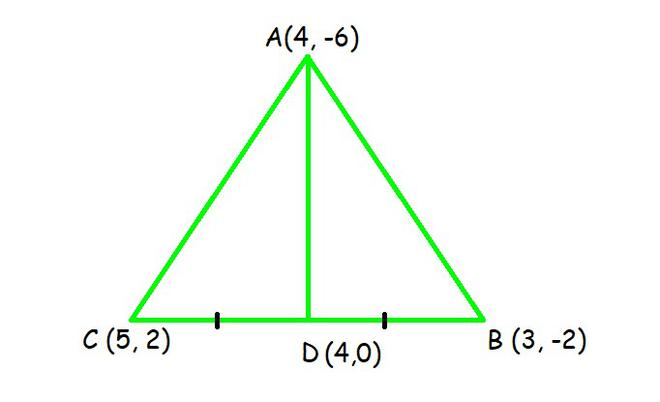Formula: Area = 1/2 [x1(y2 – y3) + x2(y3 – y1) + x3(y1 – y2)]

Now,

Area of ΔABD = 1/2 [(4)((-2) – (0)) + 3((0) – (-6)) + (4)((-6) – (-2))]

= 1/2 [-8 + 18 – 16]

= -3 square units

As we know that, area cannot be negative. So, the area of ΔABD is 3 square units.

Area of ΔACD = 1/2 [(4)(0 – (2)) + 4((2) – (-6)) + (5)((-6) – (0))]

= 1/2 [-8 + 32 – 30]

= -3 square units

As we know that, area cannot be negative. So, the area of ΔACD is 3 square units.

The area of both sides is same.

So we can say that, median AD has divided ΔABC in two triangles of equal areas.

My Personal Notes arrow_drop_up